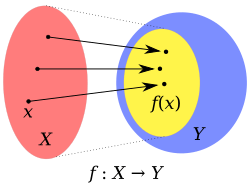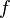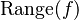# Range (mathematics) facts for kids

Kids Encyclopedia FactsImage of a function f from X to Y. The yellow oval inside Y is the range of f. Y is the Codomain of f.

In mathematics, the range is a concept which has various meanings in different topics:

• The range of a function$f$, also written as$\operatorname{ran}(f)$ or$\operatorname{Range}(f)$, is the set of elements that the function outputs.
• In statistics, the range is the difference between the lowest and highest values. For example. in {4, 6, 9, 3, 7}, the lowest value is 3, and the highest is 9, so the range is 9-3 = 6.
• In some occasions, the term "range" can also refer to the mathematical concept of interval.

## Related pagesRange (mathematics) Facts for Kids. Kiddle Encyclopedia.